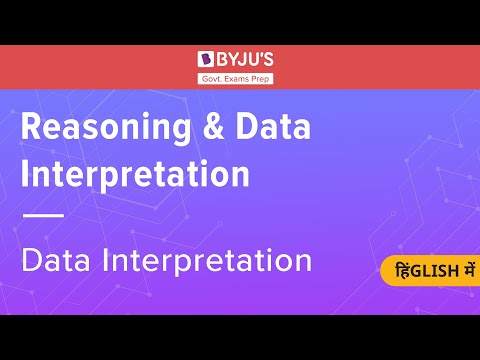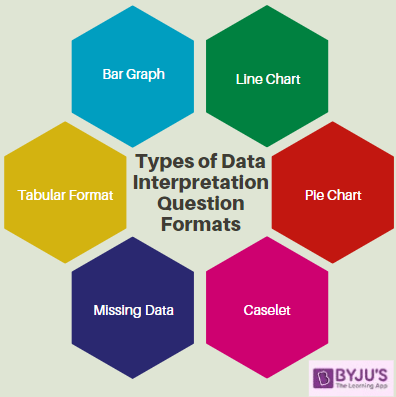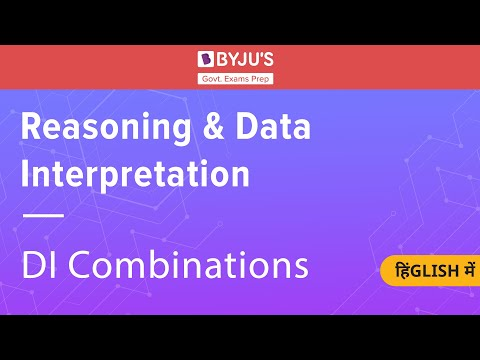# How To Prepare Data Interpretation For Bank Exams In 2023

In this article, we shall look into how to prepare Data interpretation section of Bank Exams 2023.

Data Interpretation questions have more weightage and you can see this type of question in almost every competitive bank exam question paper.

Data Interpretation (DI) is one of the most important topics in the Quantitative Aptitude section of various bank exams in the year 2023. Usually, candidates take a lot of time to solve these type of questions in bank exams.

This topic generally consists of bank exam questions related to the graph, charts and tables and some times in the form of statements too. The questions which are in the form of statements, you have to give meaningful conclusions and the questions can be from any topic such as time and distance, averages, simple interest and compound interest etc. of the Quantitative Aptitude section.

To explore the Quantitative Aptitude section in detail, check at the linked article.The data interpretation questions consist of more information part. The only key to solving these types of questions is to pick up the key points on which you can work so that you can find the answers to those questions.

Candidates can refer to the links given below and prepare the other important quantitative aptitude topics for the upcoming bank exams:

For those candidates who wish to learn about Data Interpretation from the basics, they can check the video given below:## Data Interpretation – Concept and Basics

When a piece of information is given and based on that information, questions need to be solved, such representation of data and answering questions based on it is called data interpretation.

Almost 10-15 questions in the bank prelims examination and almost 10-20 questions in the mains are asked based on data interpretation.

It must be noted that good command over the other topics included in the numerical ability section is extremely important to solve the subparts of each graph or data which is presented under the data interpretation questions.

Aspirants can check the 10 simple tricks and shortcuts to solve the questions based on quantitative aptitude at the linked article.

 Looking for sample questions based on Data Interpretation?? Refer to the links given below and ace the upcoming bank exams:

### Types of Data Interpretation Questions

Data Interpretation questions may be asked in various formats. Given below are various formats in which data interpretation questions can be asked in the bank prelims or mains exam:

1. Bar Graph – Information may be presented in the bar graph format and questions based on the given information may have to answer
2. Tabular Form – A table with the required data can be given and interpreting the data given in it, the questions may have to be answered
3. Line Chart – A line chart can represent the data in linear or line format. This is also a commonly used format to ask data interpretation questions in bank exams
4. Caselet Form – Acaselet is a paragraph form os data. No image depiction is there, the information is given in the form of paragraphs
5. Pie Chart – A pie chart is a circular representation of data. One or more pie charts may be given in a single question
6. Missing Data – Another format of data interpretation questions is missing data. A table may be given with few elements missing, candidates need to solve the questions and find the missing elements### Tips and Tricks To Solve Data Interpretation Questions

Here we bring you the complete details and the important tips on how to prepare data interpretation and how to crack DI questions easily:

1. Improve the calculations:
As we know that the Quantitative Aptitude part consists of a mathematical calculation part and this involves the calculations in solving the questions. The data interpretation section is one of the topics which need both speed and accuracy. With regular practice, you can try to solve different model questions and this will help you in improving your calculations. Slowly and steadily you will find some improvement in the level of speed and the accuracy in solving the bank exam questions.
2. Analyze the question properly:
The more data they provide in the question. the more chances are there in solving the bank exam question paper easily within less time. This is the reason why analyzing the question is more important. You should practice the different difficulty level of questions, this will give you a clear view of the different types of bank exam questions and also how much time you are taking to solve those questions.
3. Speed and accuracy:
For solving the data interpretation questions you should be more comfortable with the numbers, calculations, fractions and the percentages. This is more useful when you are solving the questions as it will take a lot of time in solving the different types of bank exam questions. Try to develop shortcut tricks for cube, square and multiplication section and the different type of approaches in solving the questions. This is where your accuracy will improve with practice.
4. Never use a calculator while practising the questions:
We all know that calculators are not allowed in any bank exam. So, try to solve the questions without the calculators if you start practising now then only you can solve the questions easily and accurately. This is to be practised not only in the case of the bank exams. Candidates who are preparing for any competitive examinations or entrance examinations should follow this rule.
5. Units:
Candidates are successful in solving the data interpretation questions but they do not pay attention to the units. This mistake we can see not only in the case of bank examinations but also in every competitive examination. So while practising just doesn’t write an answer and leave it, you should also practice writing the units, it is only then you can remember it.
6. Practice, practice, and practice:
The day-to-day bank exam preparation is more important and it is the only key to success. As we know most of the data interpretation questions are bar graphs and pie charts. We would suggest candidates to read daily newspapers, journals and magazines regularly because in some cases some DI questions are based on graphs and data related to the information given in the newspapers only.

Hence, these are some tips and the strategies on how you can prepare Data Interpretation questions for various bank examinations.
At times a combination two types of graphs/ charts may be given in a single Data Interpretation questions and to know the correct approach to solve such questions, candidates can check the video given below:### Data Interpretation – Sample Questions

Aspirants must note that since the maximum part of the quantitative aptitude section is occupied by questions from data interpretation questions, candidates must ensure that special attention is paid on this topic while preparing for the examination.

Given below are a few sample questions based on data interpretation which shall help to understand the topic even better and analyse the type of questions asked in the exam.

Direction (Q1 – Q3): Study the data given below carefully and answer the following questions:

The table given below gives the data of a clothing brand “X” which own stores in different cities in India. The monthly profit of the brand in different cities has been given in the table.

 Months Cities with their Monthly Profit Lucknow Mumbai Delhi Chandigarh Chennai January Rs. 45000 Rs. 33000 Rs. 23400 Rs. 65000 Rs. 13000 February Rs. 34500 Rs. 43000 Rs. 34560 Rs. 64500 Rs. 28000 March Rs. 33000 Rs. 56500 Rs. 43200 Rs. 12340 Rs. 66000 April Rs. 50000 Rs. 12000 Rs. 17000 Rs. 19000 Rs. 22000 May Rs. 65000 Rs. 45000 Rs. 34000 Rs. 23500 Rs. 45400

Q 1. What is the average profit earned by company X in the month of April for all the cities combined together?

1. Rs. 25000
2. Rs. 24000
3. Rs. 45000
4. Rs. 33000
5. Rs. 23000

Solution:

Average profit earned by company X in the month of April for all 5 cities combined together = (50000+12000+17000+19000+22000) / 5 = 120000 / 5 = 24000

Q 2. Which of the given cities has earned the maximum profit for the 5 months combined together?

1. Chennai
2. Mumbai
3. Lucknow
4. Chandigarh
5. Delhi

Solution:

Total Profit earned by Lucknow = Rs. (45000+34500+33000++50000+65000) = Rs. 227500

Total Profit earned by Mumbai = Rs. (33000+43000+56500+12000+45000) = Rs. 189500

Total Profit earned by Chennai = Rs. (13000+28000+66000+22000+45400) = Rs. 174400

Total Profit earned by Chandigarh = Rs. (65000+64500+12340+19000+23500) = Rs. 184340

Total Profit earned by Delhi = Rs. (23400+34560+43200+17000+34000) = Rs. 152160

Q 3. What is the difference between the maximum profit earned by company X in Lucknow and the minimum profit earned by company X in Chennai?

1. Rs. 45000
2. Rs. 52000
3. Rs. 34000
4. Rs. 65000
5. Cannot be calculated

Solution:

Maximum profit earned by company X in Lucknow was in the month of May = Rs. 65000

Minimum profit earned by company X in Chennai was in the month of January = Rs. 13000

Difference = Rs. (65000-13000) = Rs. 52000

Similar type of questions may be asked in the other data interpretation formats as well. Thus, candidates should space sufficient time to prepare the data interpretation topic to ace the upcoming bank exams.

For more information regarding the bank exam preparation of the Data Interpretation section, candidates can visit the linked article.

Aspirants willing to know more about the bank exams, preparation tips and study material and refer to BYJU’S for assistance.